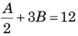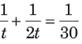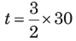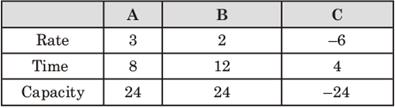Courses

# CAT Previous Year Questions: Time & Work

## 17 Questions MCQ Test Quantitative Aptitude (New) | CAT Previous Year Questions: Time & Work

Description
This mock test of CAT Previous Year Questions: Time & Work for CAT helps you for every CAT entrance exam. This contains 17 Multiple Choice Questions for CAT CAT Previous Year Questions: Time & Work (mcq) to study with solutions a complete question bank. The solved questions answers in this CAT Previous Year Questions: Time & Work quiz give you a good mix of easy questions and tough questions. CAT students definitely take this CAT Previous Year Questions: Time & Work exercise for a better result in the exam. You can find other CAT Previous Year Questions: Time & Work extra questions, long questions & short questions for CAT on EduRev as well by searching above.
QUESTION: 1

### At their usual efficiency levels, A and B together finish a task in 12 days. If A had worked half as efficiently as she usually does, and B had worked thrice as efficiently as he usually does, the task would have been completed in 9 days. How many days would A  take to finish the task if she works alone at her usual efficiency? (CAT 2019)

Solution:

Let Total work be 108 days
At normal efficiency work is done in 12 days
Let A' s day work = A & B' s day work = B
► A + B = 9 &► A + B = 9…(1)
► A + 6B = 24…(2)
Solving (1) & (2), A = 6 B = 3
At usual efficiency A will do the work in 108 / 6 = 18 days.

QUESTION: 2

### Anil alone can do a job in 20 days while Sunil alone can do it in 40 days. Anil starts the job, and after 3 days, Sunil joins him. Again, after a few more days, Bimal joins them and they together finish the job, If Bimal has done 10% of the job, then in how many days was the job done? (CAT 2019)

Solution:

Let total work be 40 units
Anil's day work = 2 unit
Sunil's 1 day work = 1 unit
For the first 3 days work done = 6  unit
Work done by Bimal = 4 unit
Work done by A and S during this time = 30 unit
Days = 30 / 3 = 10 days
Total days = 10 + 3 = 13 days

*Answer can only contain numeric values
QUESTION: 3

### A tank is fitted with pipes, some filling it and the rest draining it. All filling pipes fill at the same rate, and all draining pipes drain at the same rate. The empty tank gets completely filled in 6 hours when 6 filling and 5 draining pipes are on, but this time becomes 60 hours when 5 filling and 6 draining pipes are on. In how many hours will the empty tank get completely filled when one draining and two filling pipes are on ? (CAT 2018)

Solution:

Let the rate of each filling pipe be 'f ' litres/hr and the rate of each draining pipes be 'd' litres/hr
As per the first condition given in the question, Capacity of tank will be = (6f - 5d ) x 6 …(i)
And as per the second condition given in the question, Capacity of tank will be = (5f - 6d ) x 60 …(ii)
Since the capacity of tank is same in both the cases, on equating (i) and (ii), we have
► (6f - 5d) x 6 = (5f - 6d) x 60
► or, 6f - 5d = 50f - 60d
► or, 44f = 55d
► or, 4f = 5d
Therefore, f = 1.25d , putting this value in eq. (i),
Capacity of the tank = (6f - 5d ) x 6 = (7.5d - 5d) x 6 = 15d
When 2 filling and 1 draining pipes work simultaneously, the effective rate of filling the tank = (2f - d ),
Now putting f =1.25d in this eq. we have
Effective rate = (2.5d - d ) = 1.5d
Therefore time required to fill the empty tank = 15d / 1.5d = 10 hours.

QUESTION: 4

Humans and robots can both perform a job but at different efficiencies. Fifteen humans and five robots working together take thirty days to finish the job, whereas five humans and fifteen robots working together take sixty days to finish it. How many days will fifteen humans working together (without any robot) take to finish it?

(CAT 2018)

Solution:

Let the efficiency of humans be 'E' and the efficiency of robots be ' R'.
In the first case
Total work done = (15E + 5R) x 30 …(i)
In the second case,
Total work done = (5E + 15R) x 60 …(ii)
Since same work is finished in both the cases, so by equating (i) and (ii), we have :
► (15E + 5R) x 30 = (5E + 15R) x 60
► (15E + 5R) = (5E + 15R) x 2
► or, 15E + 5R = 10E + 30R
► or, 5E = 25R
► or, E = 5R
Putting this value of E in (I), we have
Total work = (15E + 5R) x 30 = (15E + R) x 30 = 480E
Time taken by 15 humans = 480E / 15E days = 32 days.

QUESTION: 5

When they work alone, B needs 25% more time to finish a job than A does. The two finish the job in 13 days in the following manner : A works alone till half the job is done, then A and B work together for four days, and finally B works alone to complete the remaining 5% of the job. In how many days can B alone finish the entire job?

(CAT 2018)

Solution:

Let the work completed by A in a day be 'a' units and that by B be 'b' units.
As per question : A alone works till half the work is completed. Thus, 50% of work is done and rest 50% needs to be completed.
Afterwards, A and B work together for 4 days and B works alone to complete the remaining 5% of the work. This means A and B in 4 days can complete 45% of the work.
Now suppose that the total amount of work to be done is 100 units.
► So, 4 a + 4b = 45 …(i)
Because B  needs 25% more time than A to finish a job.
► So, 1.25 x b = a …(ii)
Substituting value of a from (ii) in (i), we get
► 5b + 4b = 45
► or, 9b = 45
► or, b = 5 units/day
Hence, B alone can finish the job in 100 / 5 = 20 days.

QUESTION: 6

A tank is emptied everyday at a fixed time point, Immediately thereafter, either pump A or pump B or both start working until the tank is full. On Monday, A alone completed filling the tank at 8 pm. On Tuesday, B alone completed filling the tank at 6 pm. On Wednesday, A alone worked till 5 pm, and then B worked alone from 5 pm to 7 pm, to fill the tank. At what time was the tank filled on Thursday if both pumps were used simultaneously all along?

(CAT 2018)

Solution:

Let the tank be emptied everyday at 'T' pm. Let 'a' litres/hr' and 'b' litres/hr be the amount of water filled by pump A and pump B, respectively.
As per question, on Monday, A alone completed filling the tank at 8 pm. Therefore, the pump A worked for (8 - T ) hours.
So, the volume of the tank = a x (8 - T ) litres.
Similarly, on Tuesday, B alone completed filling the tank at 6 pm.
Therefore, the pump B worked for (6 - T ) hours.
Hence, the volume of the tank = b x (6 - T ) litres.
On Wednesday, A alone worked till 5 pm. and then B worked alone from 5 pm to 7 pm, to fill the tank.
This means pump A worked for (5 - T ) hours and pump B worked for rest of the 2 hours. Hence, the volume of the tank = a x (5 - t) + (2 x b) litres.
We can say that a x (8 - T ) = b x (6 - T ) = a x (5 - T ) + 2b …(i)
or, a x (8 - T ) = a x (5 - T ) + 2b
or, 3a = 2b…(ii)
Again from (i) a x (8 - T ) = b x (6 - T)
From (ii) we know that 3a = 2b
So, a x (8 -T ) = (3a / 2) x (6 - T)
or, T = 2
Thus, the tank gets emptied at 2 pm daily.
A alone fills the tank at 8pm, thus, A takes 6 hours and pump B alone fills the tank at 6pm thus B takes 4 hours.
Hence, working together, both can fill the tank in (6 x 4 ) / (6 + 4 ) = 2.4 hours or 2 hours and 24 minutes.

QUESTION: 7

A water tank has inlets of two types A and B. All inlets of type A when open, bring in water at the same rate. All inlets of type B, when open, bring in water at the same rate. The empty tank is completely filled in 30 minutes if 10 inlets of type A and 45 inlets of type B are open, and in 1 hour if 8 inlets of type A and 18 inlets of type B are open. In how many minutes will the empty tank get completely filled if 7 inlets of type A and 27 inlets of type B are open ?

(CAT 2018)

Solution:

Let the rate at which each inlet of type A brings water be 'a' litres per minute and from B 'b' litres per minute.
Then, as per question 30 x (10a + 45b)
= 60 x (8a + 18b)
or, 300a + 450b = 480a + 1080b
or, 180a = 630b
or, 6a = 9b, Thus, a : b = 3 : 2 or b = (2 / 3)a …(i)
Total capacity of tank = 30 x (10a + 45b) = 30[10a + 45 x (2 / 3)a ] = 1200a
When 7 inlets of type A and 27 inlets of type B are open, the total efficiency
= 7a + 27b
= 7a + 27 x (2 / 3) a = 25a
So, time taken = capacity of tank/total efficiency = 1200a / 25a = 48 minutes
Hence, 48 min is the correct answer.

QUESTION: 8

Ramesh and Ganesh can together complete a work in 16 days. After seven days of working together, Ramesh got sick and his efficiency fell by 30%. As a result, they completed the work in 17 days instead of 16 days. If Ganesh had worked alone after Ramesh got sick, in how many days would he have completed the remaining work?

(CAT 2018)

Solution:

Let the efficiency of Ramesh be R units per day and that of Ganesh be G units per day.
As per question 16 ( R + G) = 17G + 7R + ( R - 0.3R) x 10
16R + 16G = 17G + 7R + 7R
So, G = 2R, So total efficiency when both work together = R + G = R + 2R = 3R
Hence, total work = 16 x 3R = 48R
In second case, where Ramesh got sick after 7 days
Work left after 7 days = 48R - 7 (3R) = 27R
Time Ganesh would take to complete this work alone = 27R / 2R = 13.5 days

QUESTION: 9

A person can complete a job in 120 days. He works alone on Day 1. On Day 2, he is joined by another person who also can complete the job in exactly 120 days. On Day 3, they are joined by another person of equal efficiency. Like this, everyday a new person with the same efficiency joins the work. How many days are required to complete the job?

(CAT 2017)

Solution:

Let the rate of work of a person by Y units/day.
Hence, the total work = 120Y .
Given : On first day, only one person works, on the second day two people work and so on.
Hence, the work done on day 1, day 2,… will be Y ,2Y ,3Y …respectively.
Let the total number of days required to complete the work is n.
Thus sum should be equal to 120Y.
So, Y + 2Y + 3Y +…+ nY = 120Y
or, 120Y = Y x n (n - 1) / 2
or, n2 + n - 240 = 0 or (n - 15)(n + 16) = 0
So, n = 15 or n = -16
But, n = 15 is the only positive value.
Hence, it will take 15 days to complete the work.

QUESTION: 10

A tank has an inlet pipe and an outlet pipe. If the outlet pipe is closed, then the inlet pipe fills the empty tank in 8 hours. If the outlet pipe is open, then the inlet pipe fills the empty tank in 10 hours. If only the outlet pipe is open, then in how many hours the full tank becomes half-full?

(CAT 2017)

Solution:

Let the time taken by the outlet pipe to empty the tank fully when only outlet pipe is open = T hours.
Then, as per question [(1 / 8) - (1 / T )] = 1 /10
or, 1 / T = 1 / 8 - 1 /10 = 1 / 40,
therefore the outlet pipe can empty the tank fully in 40 hours.
Hence, time taken by the outlet pipe to make the tank half-full = 40 / 2 = 20 hours.

QUESTION: 11

Amal can complete a job in 10 days and Bimal can complete it in 8 days. Amal, Bimal and Kamal together complete the job in 4 days and are paid a total amount of Rs. 1000 as remuneration. If this amount is shared by them in proportion to their work, then Kamal's share, in rupees, is

(CAT 2017)

Solution:

Amal, Bimal and Kamla can together complete the job in 4 days.
Amal's contribution in 1 day =1 / 10 and Bimal's contribution = 1 / 8.
So Kamal's contribution = 1 / 4 - (1 / 8 + 1 / 10) = 1 / 40

Ratio of their work
= 1 / 10 : 1 / 8 : 1 / 40 = 4 : 5 : 1
So, amount of share for Kamal = 1000 x 1 /10 = ₹ 100

QUESTION: 12

Four two-way pipes, A,B,C and D can either fill an empty tank or drain the full tank in 4, 10, 12 and 20 minutes, respectively. All four pipes were opened simultaneously when the tank is empty. Under which of the following conditions, the tank would be half filled after 30 minutes?

(XAT 2017)

Solution:

Let the capacity of the tank
► = LCM (4 ,10,12,20) = 60
So, work done by A, B, C =15, 6, 5, 3 respectively.
Option 1 : Work Done in a minute
► = 15 - 6 - 5 - 3 = 1
So, after 30 minutes, the tank will be half filled.

QUESTION: 13

A water tank has M inlet pipes and N outlet pipes. An inlet pipe can fill the tank in 8 hours while an outlet pipe can empty the full tank in 12 hours. If all pipes are left open simultaneously, it takes 6 hours to fill the empty tank. What is the relationship between M and N?

(XAT 2016)

Solution:

As per question, M inlet pipes can fill the M / 8th part of the tank in 1 hour and N outlet pipes can empty N / 12th part of the tank in 1 hour. If all pipes are left open, in 1 hour, the tank will be filled = M / 8 - N /12 = 1 / 6 (as the tank will take 6 hours to fill). Then, 6 M - 4 N = 8, or, M = (8 + 4 N ) / 6. There will be infinite number of solutions ( N = 1,4 ,7,10,13,16 ...) but none of the above ratios are correct. For example, above equation is solvable when M = 2,N = 1 or M = 4, N = 4. But the ratios 2 : 1 (i.e., M = 2,N = 1 ) or 1 : 1 ( M = 4, N = 4 ) are not correct because the solution of the above equation will not be an integer for values like M = 4 ,N = 2 or M = 6, N = 6.

QUESTION: 14

In the marketing management course of an MBA programme, you and your roommate can complete an assignment in 30 days. If you are twice as efficient as your roommate, the time required by each to complete the assignment individually is

(IIFT 2016)

Solution:

Let your efficiency of doing some work be t days, then the efficiency of your roommate is 2t days.
So, we have,t = 45 days thus, 2t = 90 days

QUESTION: 15

Three pipes are connected to an inverted cone, with its base at the top. Two inlet pipes, A and B, are connected to the top of the cone and can fill the empty cone in 8 hours and 12 hours, respectively. The outlet pipe C, connected to the bottom, can empty a filled cone in 4 hours. When the cone is completely filled with water, all three pipes are opened. Two of the three pipes remain open for 20 hours continuously and the third pipe remains open for a lesser time. As a result, the height of the water inside the cone comes down to 50%. Which of the following options would be possible?

(XAT 2015)

Solution:

The two inlet A and B and one outlet pipe C, fill or empty the tank as per rates tabulated below :Given : Two of the pipes are kept open for 20 hours and the third pipe C for a lesser period.
The tank is initially full and finally (volume = (1 / 3) x π x r2h) the water level drops to 1 / 2 and so the volume will become (1 / 3) x π x (r / 2)2 x  h / 2 = 1 / 8 {(1 / 3) x π x r2h )}, i.e., 1 / 8th of the original volume.
This means 7 / 8th volume of water must have drained out.
From the table above, we can see that net rate of emptying the tank = 3 + 2 - 6 = 1 liter per hr.
So, to drain the full tank out, when all the pipes are open, it will take 24 hrs.
So, to drain 7 / 8th tank = 7 x 24 / 8 = 21 hrs.
Since, the 7 / 8th water drained out in 20 hrs only, so one of the two inlet pipes must have opened for lesser time. Assuming that if all the three pipes were open for all 20 hrs, we can see that 1 extra unit was required to be drained or the water was not filled by closing one of the inlet pipes a little early.
From the table, we can see that pipe B if opened for 1 / 2 hrs less, will fill the water by 1 litre less.
So, B works for 19.5 hrs only.

QUESTION: 16

A tank is connected with both inlet pipes and outlet pipes. Individually, an inlet pipe can fill the tank in 7 hours and an outlet pipe can empty it in 5 hours. If all the pipes are kept open, it takes exactly 7 hours for a completely filled-in tank to empty. If the total number of pipes connected to the tank is 11, how many of these are inlet pipes?

(IIFT 2015)

Solution:

Let there be i and o inlet and outlet pipes respectively.
∴ i + o = 11…(i)
Assume that the capacity of the tank is 35 units.
So, inlet pipe fills 5 units and the outlet pipes empties 7 units of the tank in one hour.
The completely filled tank empties in 7 hours.
∴ 7o - 5i = 5…(ii)
So, now we solve the equations, and get i and o and i = 6.

QUESTION: 17

Three carpenters P,Q and R are entrusted with office furniture work. P can do a job in 42 days. If Q is 26% more efficient than P, and R is 50% more efficient than Q, then Q and R together can finish the job in approximately :

(IIFT 2015)

Solution:

Let P do x units of work in one day.
∴ so, total work = 42x
So, Q and R do 1.26x and (1.26 x 1.5 =)1.89x units of work in one day, respectively.
∴ Total work done by Q and R in one day = 1.26x + 1.89x = 3.15x
So, Q and R can finish the work in (42x / 3.15x ≈) 13 days.